Courses

# Current MCQ Level - 1

## 40 Questions MCQ Test Exclusive Video Lectures of Class 12 Physics by Experts | Current MCQ Level - 1

Description
This mock test of Current MCQ Level - 1 for Class 12 helps you for every Class 12 entrance exam. This contains 40 Multiple Choice Questions for Class 12 Current MCQ Level - 1 (mcq) to study with solutions a complete question bank. The solved questions answers in this Current MCQ Level - 1 quiz give you a good mix of easy questions and tough questions. Class 12 students definitely take this Current MCQ Level - 1 exercise for a better result in the exam. You can find other Current MCQ Level - 1 extra questions, long questions & short questions for Class 12 on EduRev as well by searching above.
QUESTION: 1

Solution:
QUESTION: 2

Solution:
QUESTION: 3

### In the circuit shown in Fig. switch S is closed at time t = 0, Let l1 and l2 be the currents at any finite time t, then the ratio I1 / I2.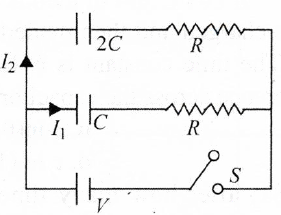Solution:
QUESTION: 4

In the circuit shown in fig. C1 = 2C2. Initially, capacitor C1 is charged to a potential of V. The current in the circuit just after the switch S is closed is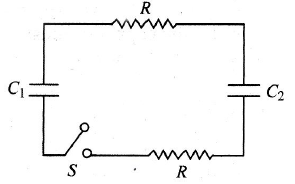Solution:
QUESTION: 5

ln the circuit given in Fig. switch S is at position 1 for long time. Find the total heat generated in resistor of resistance (2r), when the switch S is shifted from 1 to position 2.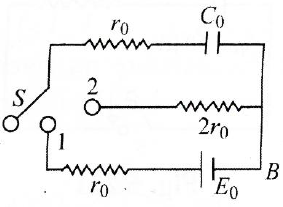Solution:
QUESTION: 6

In the circuit in Fig., if no current flows through the galvanometer when the key k is closed, the bridge is balanced. The balancing condition for bridge is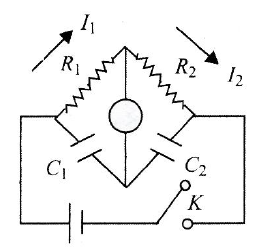Solution:
QUESTION: 7

A capacitor of capacitance C has charge Q. It is connected to an identical capacitor through a resistance. The heat produced in the resistance is:

Solution:
QUESTION: 8

In the circuit shown in Fig. the cell is ideal with e.m.f = 15 V. Each resistance is of 3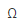. The potential difference across the capacitor in steady state is: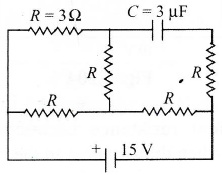Solution:
QUESTION: 9

A straight conductor of uniform cross section carries a time varying current which varies at the rate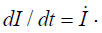If s is the specific charge that is carried by each charge carrier of the conductor and l is the length of the conductor, then the total force experienced by all the charge carriers per unit length of the conductor due to their drift velocities only is

Solution:
QUESTION: 10

A capacitor is charged to a certain potential and then allowed to discharge through a resistance R. The ratio of charge on the capacitor to current in the circuit

Solution:
QUESTION: 11

Two capacitor C1 and C2 (C1 > C2) are charged separately to same potential. Now they are allowed to discharge through similar resistors. Initial rate of discharging will be

Solution:
QUESTION: 12

In the circuit shown in the diagram, E is the e.m.f of the cell, connected to two resistances, each of magnitude R and a capacitor of capacitance C as shown in the diagram. If the switch key K is connected at time t = 0, the growth of potential V across the capacitor will be correctly given by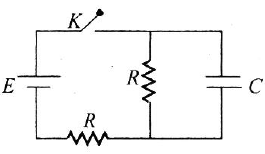Solution:
QUESTION: 13

The plates of capacitor are charged to a potential difference of 320 V and then connected across a resistor. If potential difference between plates of capacitor becomes 240 V after 1 s, then potential difference after 2 and 3 s will be

Solution:
QUESTION: 14

A 4 μF capacitor and a resistance of 2.5 x 106are connected in series with a battery of 12 V. Time after which potential difference across the capacitor is 3 times of potential difference across the resistor is

Solution:
QUESTION: 15

A capacitor is charged steadily from a DC source. Correct variation of potential difference across the plates of capacitor with charge on the plates of capacitor is:

Solution:
QUESTION: 16

A capacitor is made to discharge across a pure resistor. A plot of log I versus t (l = instantaneous current and t = instantaneous time) is recorded as shown in graph 1. The same experiment is repeated with a change and graph 2 is observed. Then,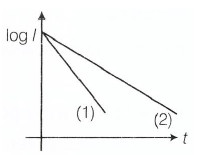Solution:
QUESTION: 17

Three identical capacitors are charged by connecting them in parallel across a battery of V volt. They are then allowed to discharge via resistor R1, R2 and R3. Here, Q charge versus t time graphs are shown. Then,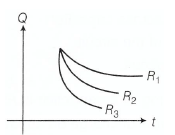Solution:
QUESTION: 18

For the circuit shown in figure below, At t = 0, switch is closed, the initial current through resistor and final charge on capacitor are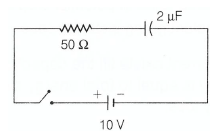Solution:
QUESTION: 19

For the given circuit shown in figure below, Time constant is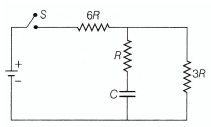Solution:
QUESTION: 20

During charging of capacitor in the circuit shown,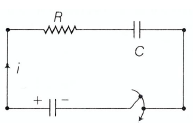Circuit current versus time graph is

Solution:
QUESTION: 21

During discharging of a capacitor via a resistor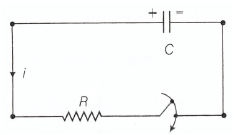Circuit current versus with time as

Solution:
QUESTION: 22

If t indicates instantaneous time, then t\RC

Solution:
QUESTION: 23

In a capacitor shown in the circuit is charged to 5V and left in the circuit in 12 S. The charge on the capacitor will becomes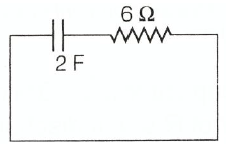Solution:
QUESTION: 24

How will the reading in the ammeter A of Fig. be affected if another identical bulb Q is connected in parallel to P as shown. The voltage in the mains is maintained at a constant value.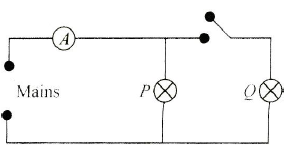Solution:
QUESTION: 25

In the circuit shown in Fig., the reading of the ammeter is (assume internal resistance of the battery be to zero)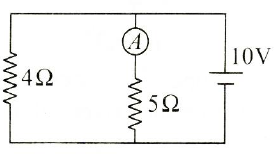Solution:
QUESTION: 26

In the circuit shown in Fig. resistors Xand Y, each with resistance R are connected to a 6V battery of negligible internal resistance. A voltmeter, also of resistance R, is connected across Y.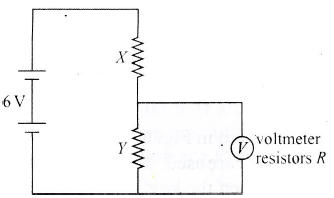What is the reading of the voltmeter?

Solution:
QUESTION: 27

In the shown arrangement of the experiment of a meter bridge, if AC corresponding to null deflection of galvanometer is x, what would be its value if the radius of the wire AB is doubled?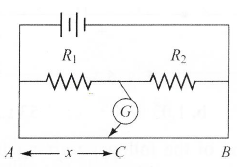Solution:
QUESTION: 28

The length of a wire of a potentiometer is 100 cm, and the e.m.f. of its standard cell is E volt. It is employed to measure the e.m.f. of a battery whose internal resistance is 0.5. If the balance point is obtained at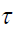= 30 cm from the positive end, e.m.f. of the battery is

Solution:
QUESTION: 29

In a metre bridge experiment, null point is obtained at 20 cm from one end of the wire when resistance X is balanced against another resistance Y. If X < Y, then where will be the new position of the null point from the same end, if one decides to balance a resistance of 4X against Y?

Solution:
QUESTION: 30

In the circuit shown in Fig., the galvanometer G shows zero deflection. If the batteries A and B have negligible internal resistance, the value of the resistor R will be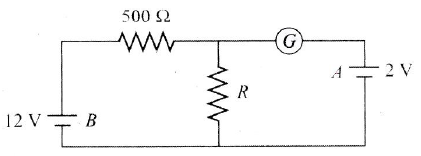Solution:
QUESTION: 31

In a potentiometer experiment, the balancing with a cell is length 240 cm. On shunting the cell with a resistance of 2, the balancing length becomes 120 cm. The internal resistance of the cell is

Solution:
QUESTION: 32

If the experiment of Wheatstone bridge, the positions of cells and galvanometer are interchanged, then the balance points will

Solution:
QUESTION: 33

Sensitivity of the potentiometer can be increased by

Solution:
QUESTION: 34

The resistance of a galvanometer is 10. It gives fullscale deflection when 1 mA current is passed. The resistance connected in series for converting it into a voltmeter of 2.5 V will be

Solution:
QUESTION: 35

A milliammeter of range 10 mA has a coil of resistance 1.To use it as an ammeter of range 1 A, the required shunt must have a resistance of

Solution:
QUESTION: 36

Figure shows a circuit which may be used to compare the resistance R of an unknown resistor with a 100standard. The distancesi from one end of the potentiometer slider wire to the balance point are 400 mm and 588 mm when X is connected to Y and Z, respectively. The length of the slide wire is 1.00m. What is the value of resistance R?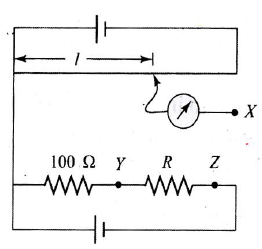Solution:
QUESTION: 37

Figure shows a simple potentiometer circuit for measuring a small e.m.f. produced by a thermocouple. The metre wire PQ has a resistance of 5and the driver cell has an e.m.f. of 2.00 V. If a balance points is obtained 0.600 m along PQ when measuring an e.m.f. of 6.00 mV, what is the value of resistance R?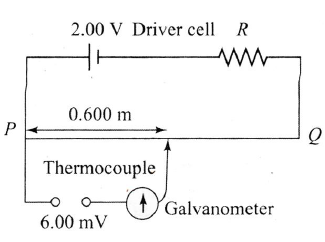Solution:
QUESTION: 38

If a shunt of 1/10th of the coil resistance is applied to a movingt coil galvanometer, its sensitivity becomes

Solution:
QUESTION: 39

In Fig. when an ideal voltmeter is connected across 4000 W resistance, it reads 30 V. If the voltmeter is connected across 3000resistance, it will read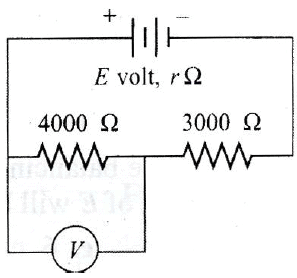Solution:
QUESTION: 40

Three bulbs of 40W, 60W and 100W are arranged in series with 220V, which bulb has minimum resistance?

Solution: